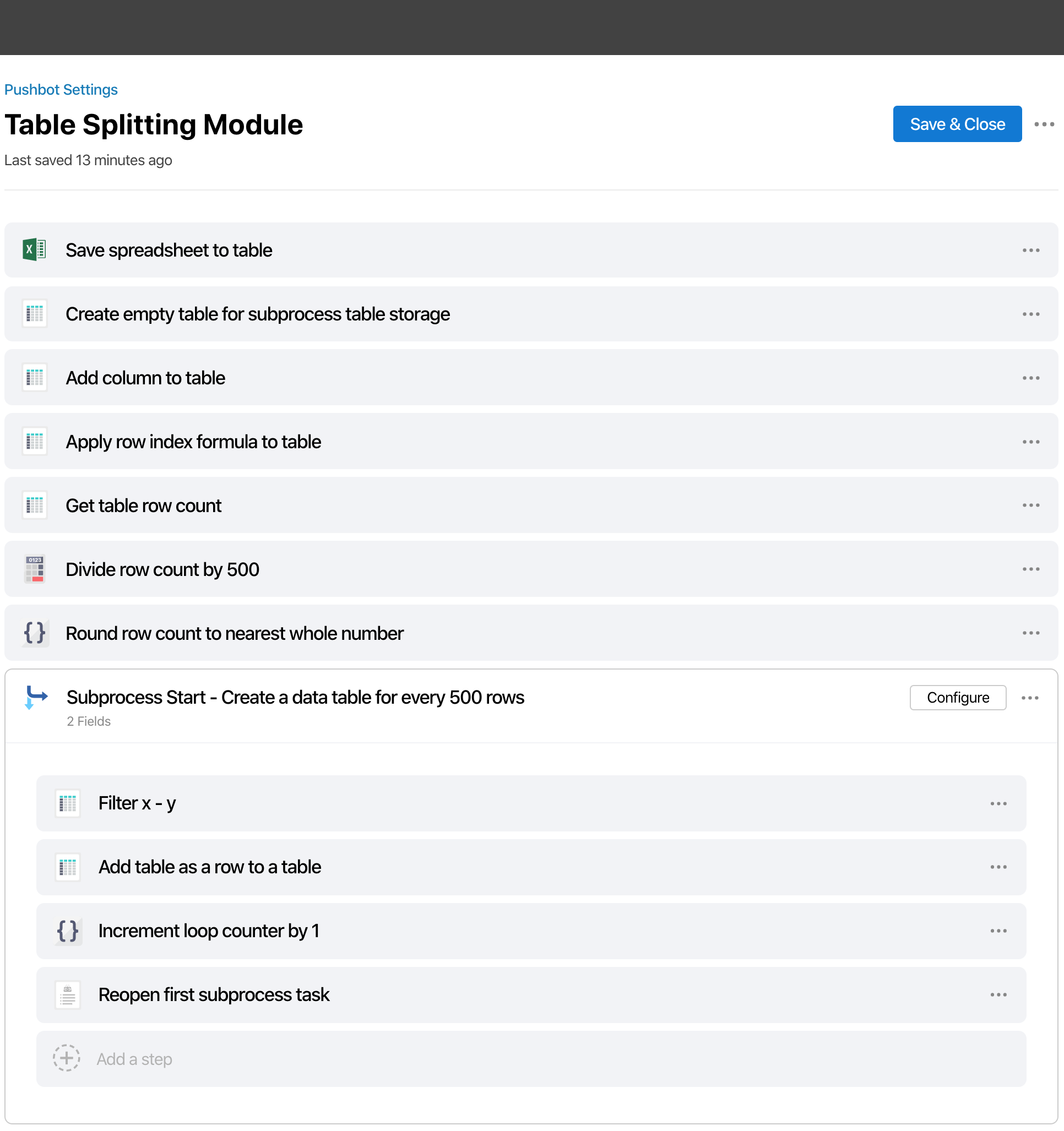# Split Table Every 500 Rows

edited April 2

I shared my initial setup image below for feedback on this building approach.

Questions:

• Have you encountered the need to split a table by a set row count?
• Have you used a similar building approach or a different one?

Background:
For every 500 rows in the trigger attachment spreadsheet, I need 1 data table. 10k rows is the max spreadsheet row count, totaling 20 output tables at most in some cases.

1. First we save the trigger attachment file to a data table, then apply a row index table formula.
2. Second, with some basic math and rounding, we identify the number of times we must copy 500 rows.
3. Third, we start a subprocess for looping.

Looping Subprocess:
In this loop, we use a dynamic filter to filter the trigger attachment table. (First loop filters rows 1-500, second loop filters rows 500-1000, etc. until the loop completes.) Every time we produce a 500 row table, we save it to a separate data table. We then increment a loop counter field, and re-run the loop. Once the loop completes, the output should be a data table that contains all the data tables output from the loops.